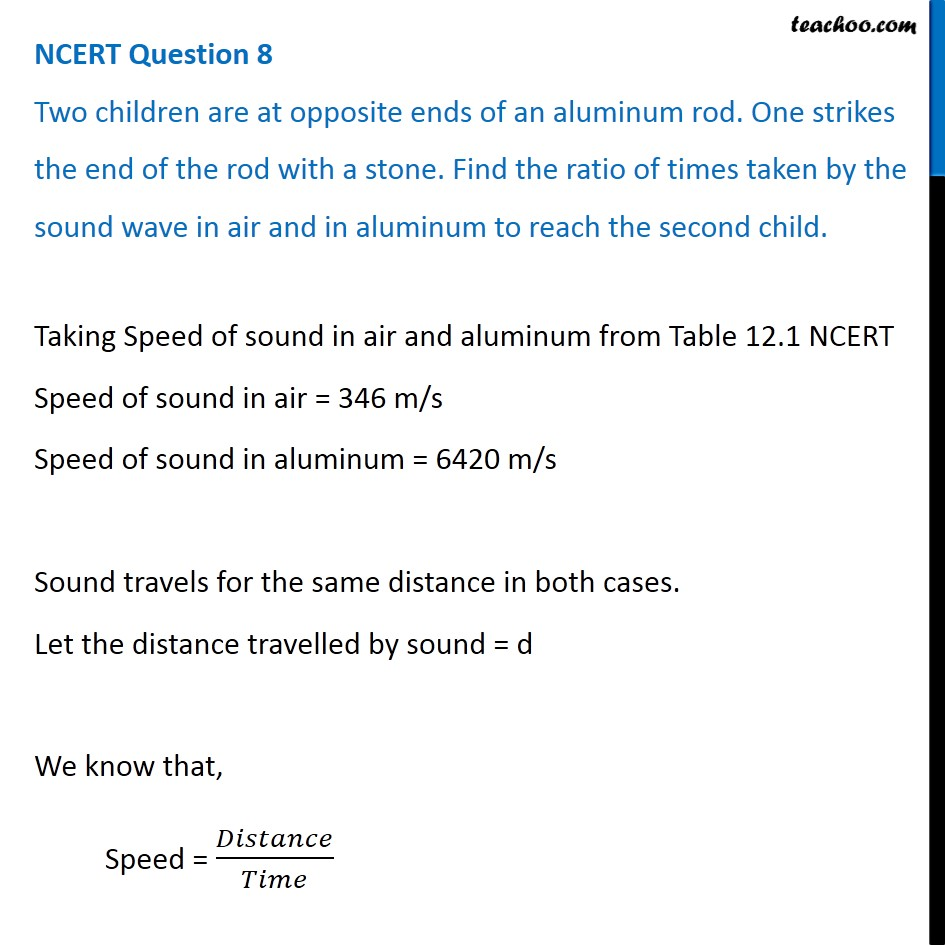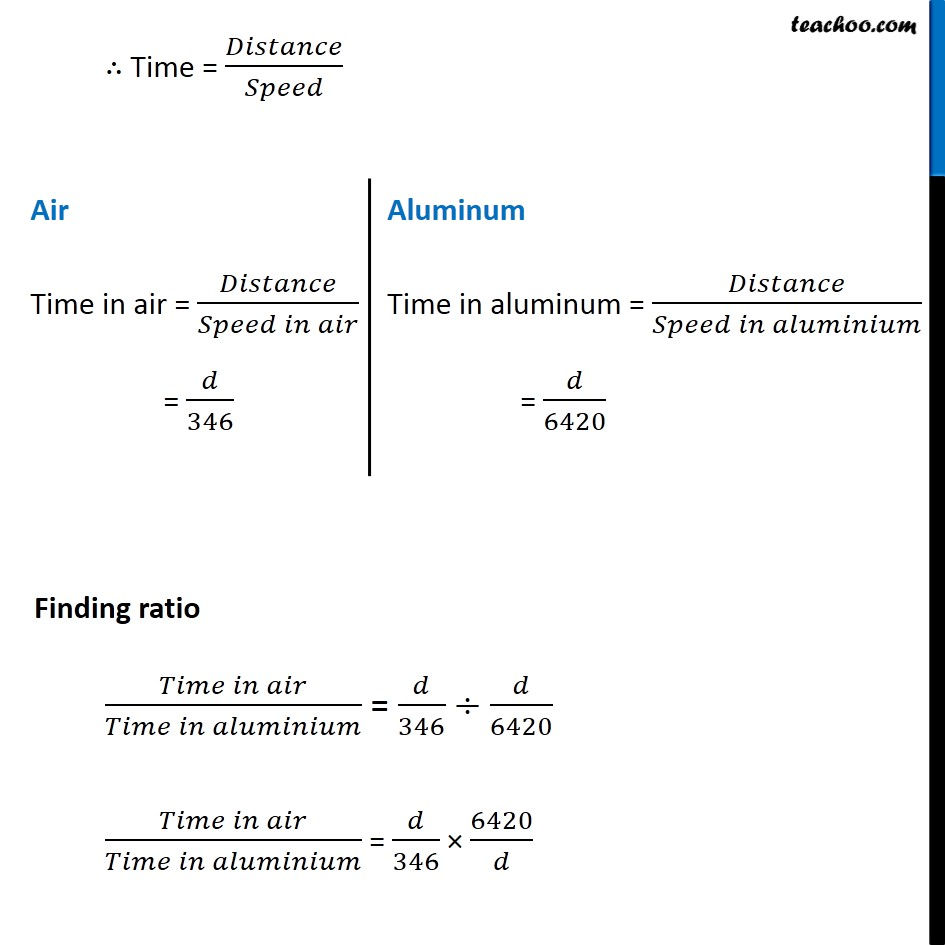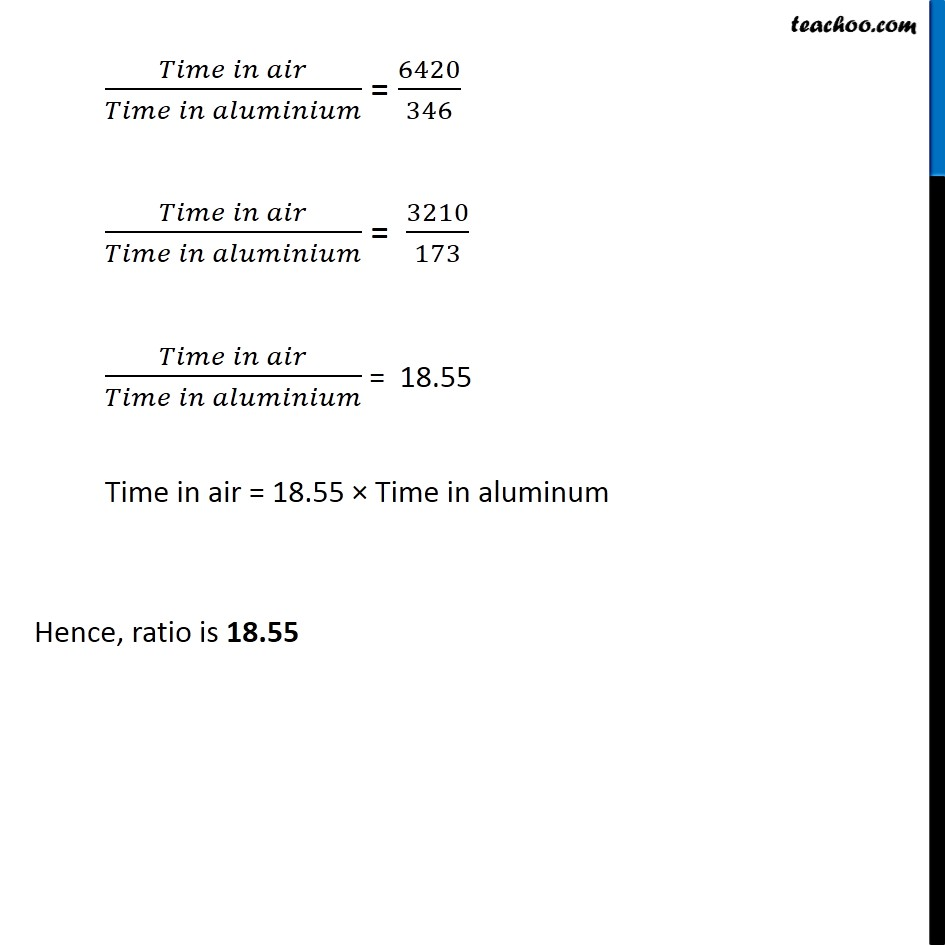NCERT Questions

Class 9
Chapter 12 Class 9 - SoundLearn in your speed, with individual attention - Teachoo Maths 1-on-1 Class

### Transcript

NCERT Question 8 Two children are at opposite ends of an aluminum rod. One strikes the end of the rod with a stone. Find the ratio of times taken by the sound wave in air and in aluminum to reach the second child. Taking Speed of sound in air and aluminum from Table 12.1 NCERT Speed of sound in air = 346 m/s Speed of sound in aluminum = 6420 m/s Sound travels for the same distance in both cases. Let the distance travelled by sound = d We know that, Speed = 𝐷𝑖𝑠𝑡𝑎𝑛𝑐𝑒/𝑇𝑖𝑚𝑒 ∴ Time = 𝐷𝑖𝑠𝑡𝑎𝑛𝑐𝑒/𝑆𝑝𝑒𝑒𝑑 Air Time in air = 𝐷𝑖𝑠𝑡𝑎𝑛𝑐𝑒/(𝑆𝑝𝑒𝑒𝑑 𝑖𝑛 𝑎𝑖𝑟) = 𝑑/346 Aluminum Time in aluminum = 𝐷𝑖𝑠𝑡𝑎𝑛𝑐𝑒/(𝑆𝑝𝑒𝑒𝑑 𝑖𝑛 𝑎𝑙𝑢𝑚𝑖𝑛𝑖𝑢𝑚) = 𝑑/6420 Finding ratio (𝑇𝑖𝑚𝑒 𝑖𝑛 𝑎𝑖𝑟)/(𝑇𝑖𝑚𝑒 𝑖𝑛 𝑎𝑙𝑢𝑚𝑖𝑛𝑖𝑢𝑚) = 𝑑/346 ÷𝑑/6420 (𝑇𝑖𝑚𝑒 𝑖𝑛 𝑎𝑖𝑟)/(𝑇𝑖𝑚𝑒 𝑖𝑛 𝑎𝑙𝑢𝑚𝑖𝑛𝑖𝑢𝑚) = 𝑑/346 × 6420/𝑑 (𝑇𝑖𝑚𝑒 𝑖𝑛 𝑎𝑖𝑟)/(𝑇𝑖𝑚𝑒 𝑖𝑛 𝑎𝑙𝑢𝑚𝑖𝑛𝑖𝑢𝑚) = 6420/346 (𝑇𝑖𝑚𝑒 𝑖𝑛 𝑎𝑖𝑟)/(𝑇𝑖𝑚𝑒 𝑖𝑛 𝑎𝑙𝑢𝑚𝑖𝑛𝑖𝑢𝑚) = 3210/173 (𝑇𝑖𝑚𝑒 𝑖𝑛 𝑎𝑖𝑟)/(𝑇𝑖𝑚𝑒 𝑖𝑛 𝑎𝑙𝑢𝑚𝑖𝑛𝑖𝑢𝑚) = 18.55 Time in air = 18.55 × Time in aluminum Hence, ratio is 18.55